# array

Create array of PCB stack objects

## Syntax

``pcbArr = array(pcbObj,'linear')``
``pcbArr = array(pcbObj,'rectangular')``
``pcbArr = array(pcbObj,'circular')``
``pcbArr = array(___,Name,Value)``

## Description

example

````pcbArr = array(pcbObj,'linear')` creates a default linear array of the input PCB stack object `pcbObj`. The default linear array has with two elements and element spacing of 2 meters.```

example

````pcbArr = array(pcbObj,'rectangular')` creates a default rectangular array of the input PCB stack object. The default rectangular array is a 2-by-2 array with row and column spacing of 2 meters.```

example

````pcbArr = array(pcbObj,'circular')` creates a default circular array of the input PCB stack object. The default circular array has six elements with a radius of 1 meter and an offset angle of 0 degrees.```
````pcbArr = array(___,Name,Value)` updates the array using one or more name-value pairs. For example, ```p = array(pcbObj,'linear','NumElements',5)``` creates a linear array of `pcbObj` PCB stack object with five elements.```

## Examples

collapse all

Create a PCB antenna from a circular patch microstrip antenna using the `pcbStack `object.

```ant = patchMicrostripCircular('Substrate',dielectric('FR4')); pcbAnt = pcbStack(ant); show(pcbAnt)```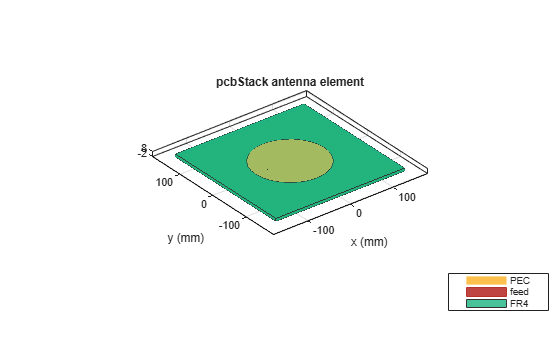Create a linear array of the antenna using the `array `function.

```pcbArr = array(pcbAnt,'linear','NumElements',5,'ElementSpacing',0.2); show(pcbArr)```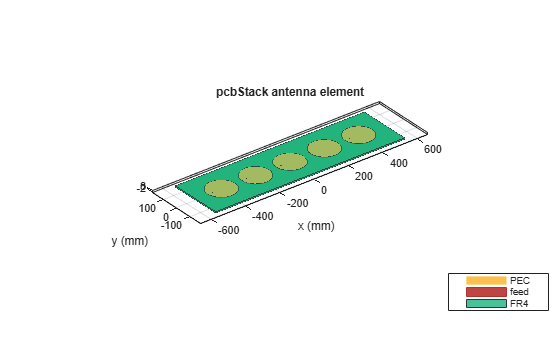Create a PCB antenna using pcbStack object

`pcbAnt = pcbStack`
```pcbAnt = pcbStack with properties: Name: 'MyPCB' Revision: 'v1.0' BoardShape: [1x1 antenna.Rectangle] BoardThickness: 0.0100 Layers: {[1x1 antenna.Rectangle] [1x1 antenna.Rectangle]} FeedLocations: [-0.0187 0 1 2] FeedDiameter: 1.0000e-03 ViaLocations: [] ViaDiameter: [] FeedViaModel: 'strip' FeedVoltage: 1 FeedPhase: 0 Conductor: [1x1 metal] Tilt: 0 TiltAxis: [1 0 0] Load: [1x1 lumpedElement] ```
```pcbAnt.Layers{1} = pcbAnt.Layers{1} - antenna.Rectangle('Length',7e-3,'Width',7e-3); pcbAnt.Layers{1} = pcbAnt.Layers{1} - antenna.Circle('Radius',5e-3,'Center',[20e-3,0]); d = dielectric('FR4'); d.Thickness = pcbAnt.BoardThickness; pcbAnt.Layers{2} = d; pcbAnt.Layers{3} = antenna.Rectangle('Length',0.15,'Width',0.075); pcbAnt.FeedLocations(end) = 3; show(pcbAnt)```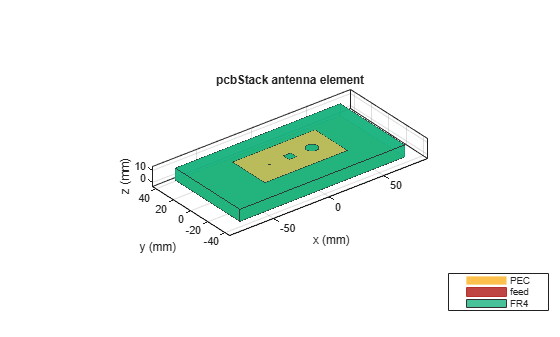Create a 4x4 rectangular array of the PCB antenna.

```pcbArr = array(pcbAnt,'rectangular','Size',[4 4],'ColumnSpacing',0.1,'RowSpacing',0.2); show(pcbArr)```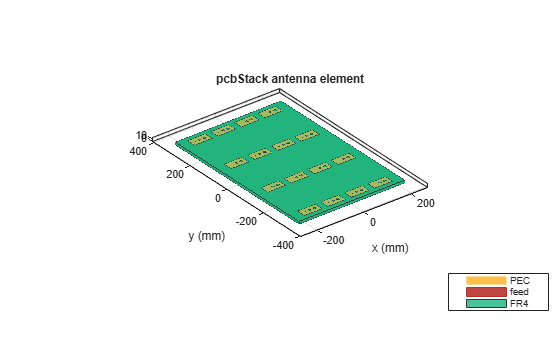Create a PCB antenna from a triangular patch microstrip antenna using the `pcbStack` object.

```ant = patchMicrostripTriangular('Substrate',dielectric('FR4')); pcbAnt = pcbStack(ant);```

Create a circular array of the PCB antenna.

```pcbArr = array(pcbAnt,'circular','Radius',0.03); show(pcbArr)```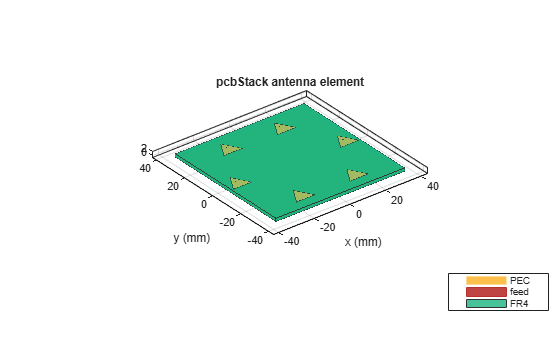## Input Arguments

collapse all

PCB antenna, specified as a `pcbStack` object.

### Name-Value Arguments

Example: `'NumElements',4`

Specify optional comma-separated pairs of `Name, Value` pair arguments. `Name` is the argument name and `Value` is the corresponding value. `Name` must appear inside single quotes (`''`). You can specify several name and value pair arguments in any order as `Name1, Value1, ..., NameN, ValueN`.

Linear Array

collapse all

Number of the antenna elements in the array, specified as a positive integer.

Example: `'NumElements',4`

Spacing between the antenna elements, specified as a positive scalar or positive vector in meters. By default, the dipole elements are spaced 2 meters apart. Use scalar for uniform and vector for non uniform spacing between the antenna elements.

Example: `'ElementSpacing',3`

Data Types: `double`

Rectangular Array

collapse all

Number of the antenna elements in the row and column of the array, specified as a two-element vector.

Example: `'Size',[4 4]`

Row spacing between the two antenna elements, specified as a scalar or vector in meters. By default, the antenna elements are spaced 2 meters apart. Use scalar for uniform and vector for non uniform spacing between the antenna elements.

Example: `'RowSpacing',0.1`

Data Types: `double`

Column spacing between the two antenna elements, specified as a positive scalar or positive vector in meters. By default, the antenna elements are spaced 2 meters apart. Use scalar for uniform and vector for non uniform spacing between the antenna elements.

Example: `'ColumnSpacing',0.1`

Data Types: `double`

Circular Array

collapse all

Number of elements in the array, specified as a positive integer. The elements in the array are arranged along the X-axis.

Example: `'NumElements',4`

Data Types: `double`

Radius of the array, specified as a positive scalar in meters.

Example: `'Radius',0.4`

Data Types: `double`

Offset angle for the first element in the array, specified as a real scalar in degrees.

Example: `'AngleOffset',8`

Data Types: `double`

## Output Arguments

collapse all

Array of the PCB antenna elements, returned as a `pcbStack` object.

## Version History

Introduced in R2021a